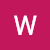# Implementation of Principal Component Analysis(PCA) in K Means Clustering

## A beginner’s approach to apply PCA using 2 components to a K Means clustering algorithm using Python and its libraries.

Prerequisites

This article assumes that you are familiar with the basic theory behind PCA, K Means Algorithm and know Python programming language.

K Means clustering is one of the simplest yet efficient unsupervised algorithms. First let us have a brief description of what this algorithm does.

K Means Algorithm
Suppose we have a dataset with two features x1 and x2. This is unlabelled data and our objective is to find K number of groups or “clusters” which are similar to each other. Suppose our training set looks like this :-• tech barbie •